# Right Angle

In this section, we will explore the world of the right angles. By finding answers to what is a sphere, how to calculate it, and discovering interesting right angle facts, the topic will be clear as a crystal.

Before we get started, let’s see what Sam has recently come across.

After observing the angle made by clock hands at 3:00 pm, one-fourth piece of a pizza, and the corners of a room, Sam noticed that right angles are all around us at several places. Do you feel the same?

A vertical and horizontal line makes the most common right angles.

Also, diagonal lines intersecting each other form a right angle.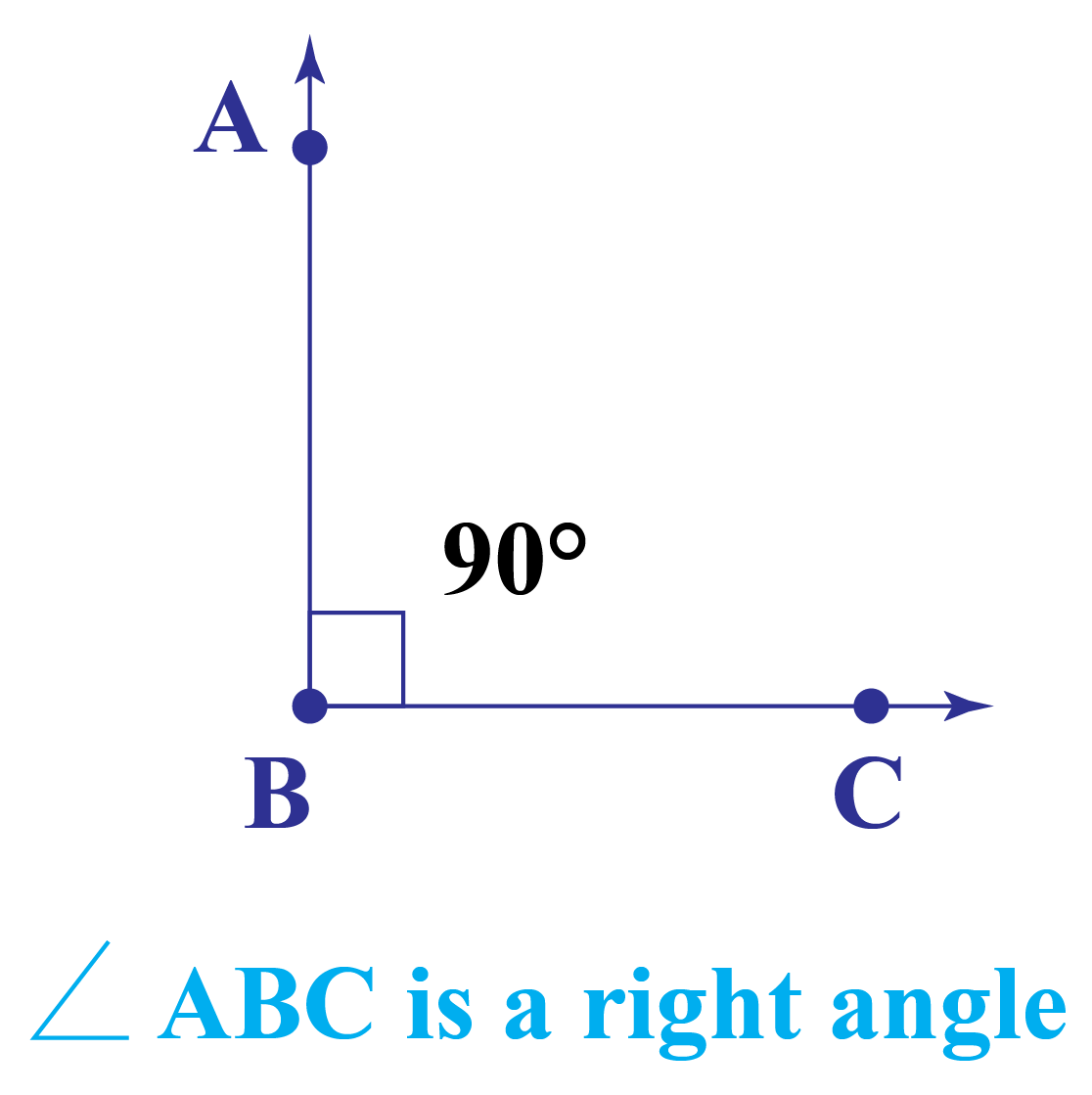Let's begin our journey and learn the amazing properties of right angles.

## Lesson Plan

 1 What Is a Right Angle? 2 Solved Examples on Right Angle 3 Interactive Questions on Right Angle

## What Is a Right Angle?

Right angle definition: A right angle is an angle with a value equal to 90 degrees.

It plays an important role in areas of mathematics such as geometry and trigonometry.
When two straight lines intersect each other at 90˚ or are perpendicular to each other at the intersection, they form a right angle. This is all about the right angle definition.

A right angle is represented by the symbol ∟.

## The Shape of a Right Angle

A right angle will look like the alphabet L.

Use the interactive simulation given and see how the right angle can be constructed using a protractor or a compass.

### Right Angle Examples in Real Life

Examples of right angles in shapes that are available around us are:

• Edges of a door.
• Four edges of television.
• 9:30 of the clock.
• Corner of the chair.

Get an idea of the right angles in shapes around us from the picture given below:

These shapes are the most commonly used for giving right angle examples.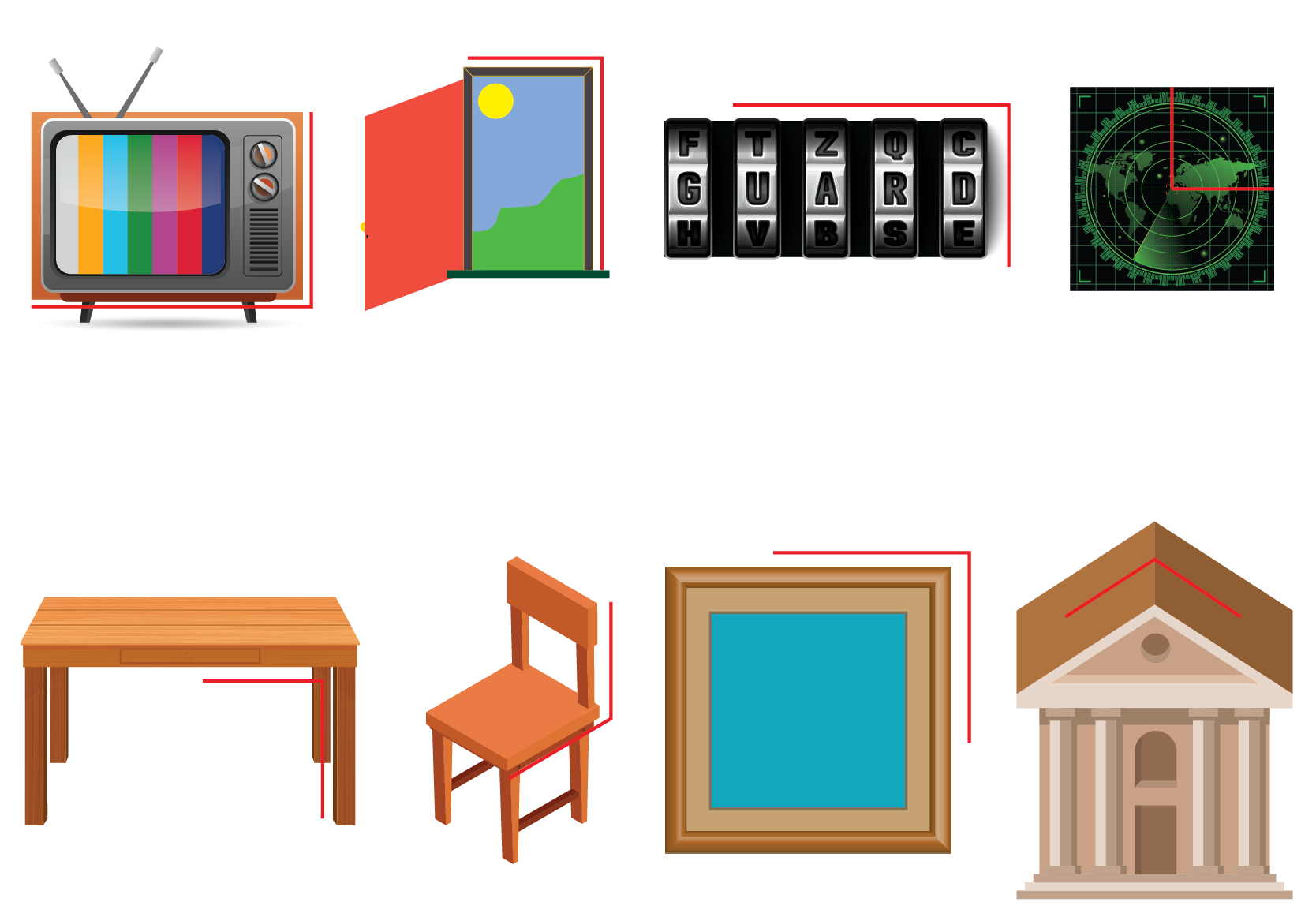Can you find a few more examples of right angles in shapes that are available around you?

## Right Angle Calculator

Some of the devices used for measuring the right angle are protractor, the set squares, try square, etc. By playing around with the simulation given below, you can see how it's done.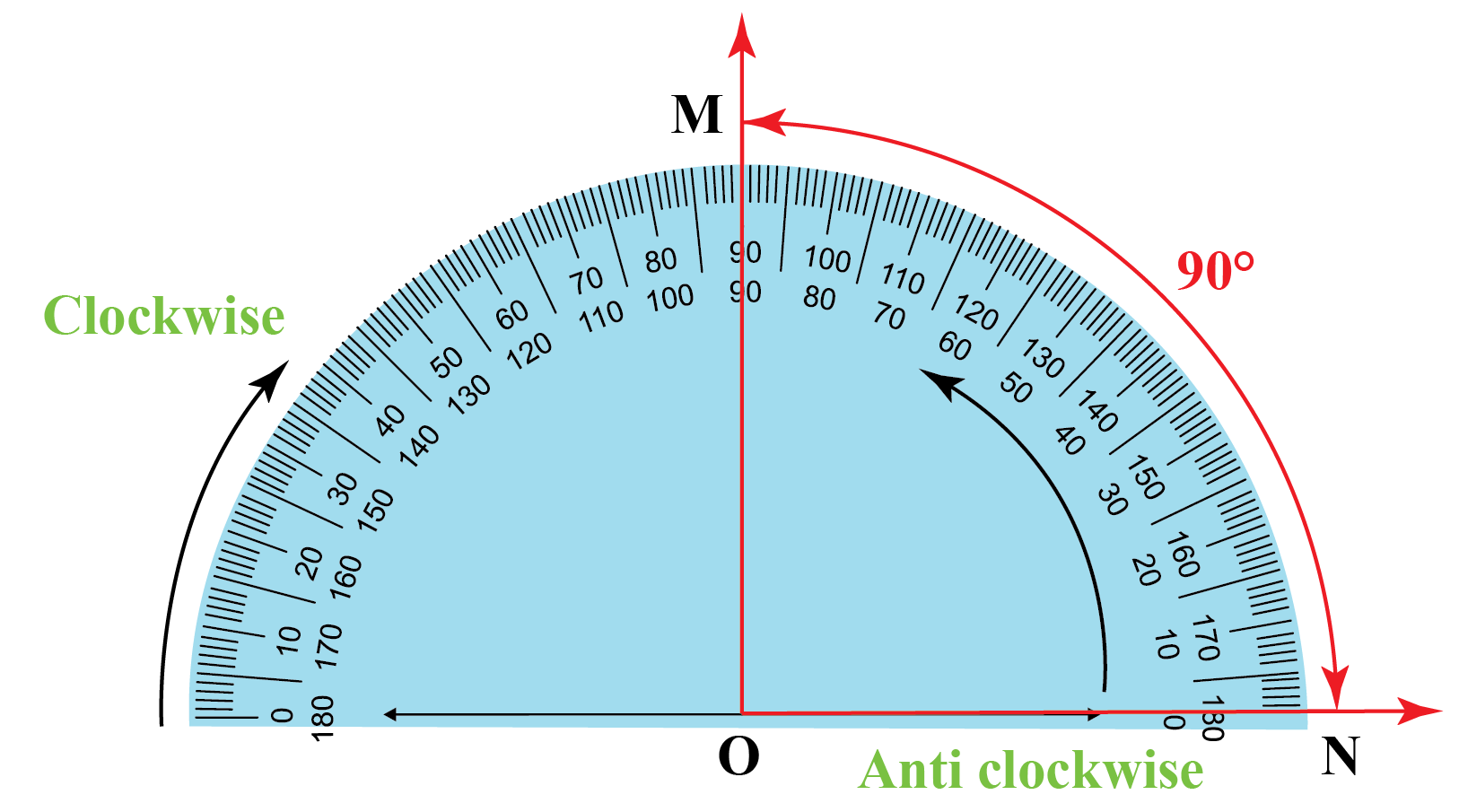Protractor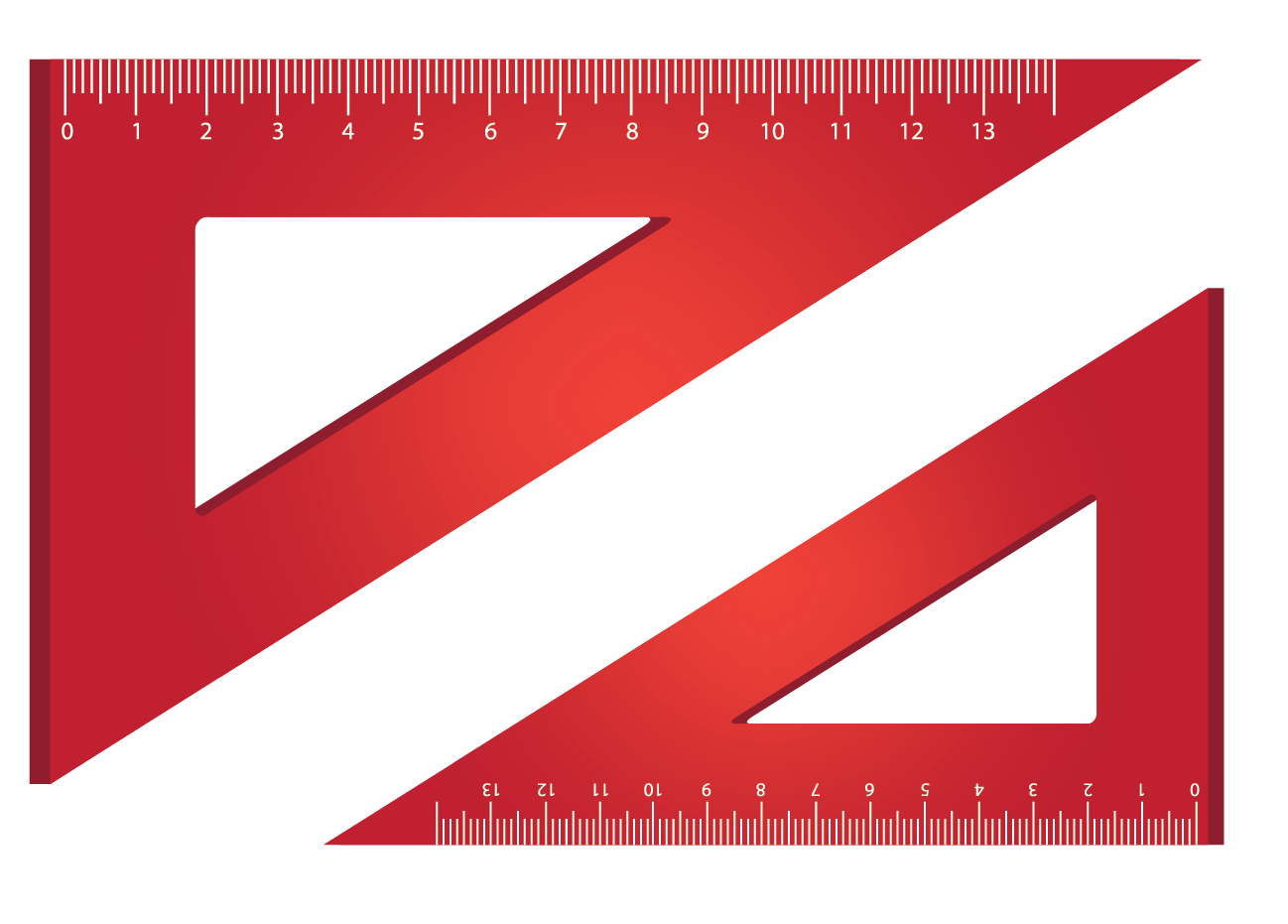The Set-Squares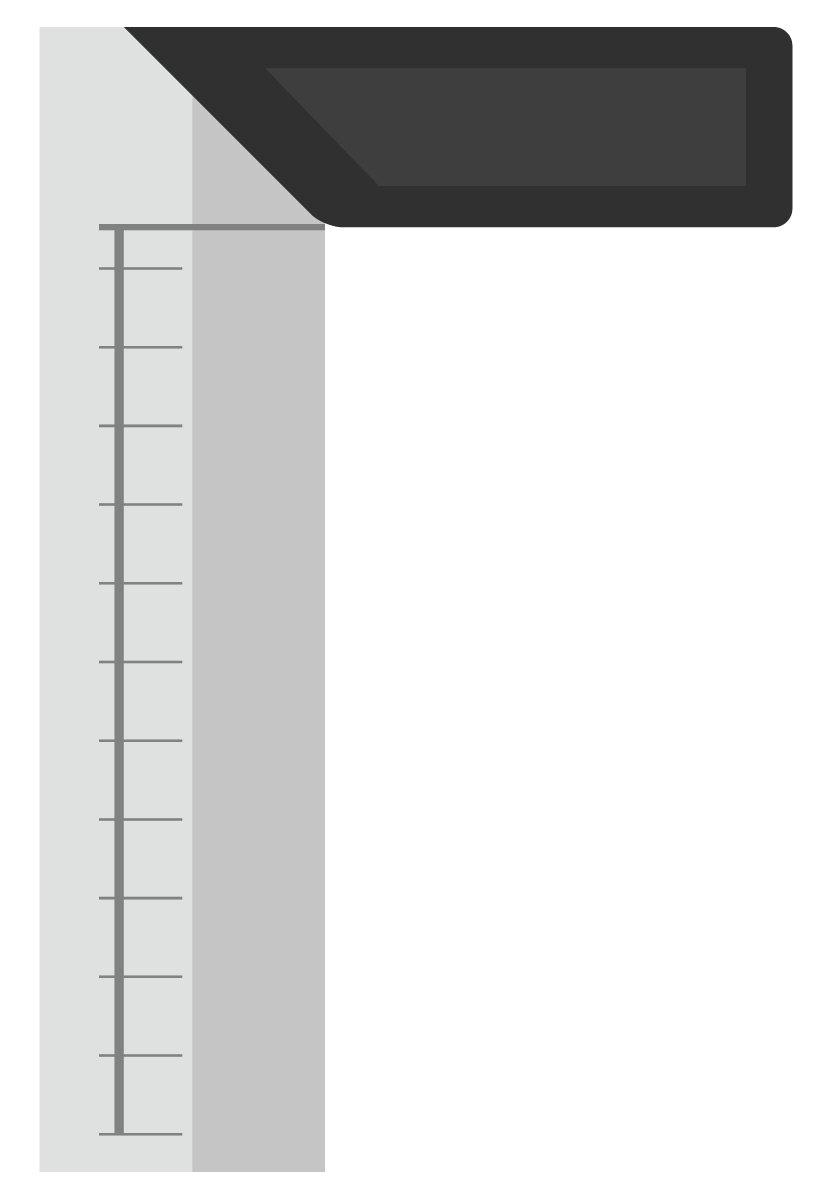Try Square

## Types of Angles

There are six different types of angles, which are as follows:

An acute angle is less than 90°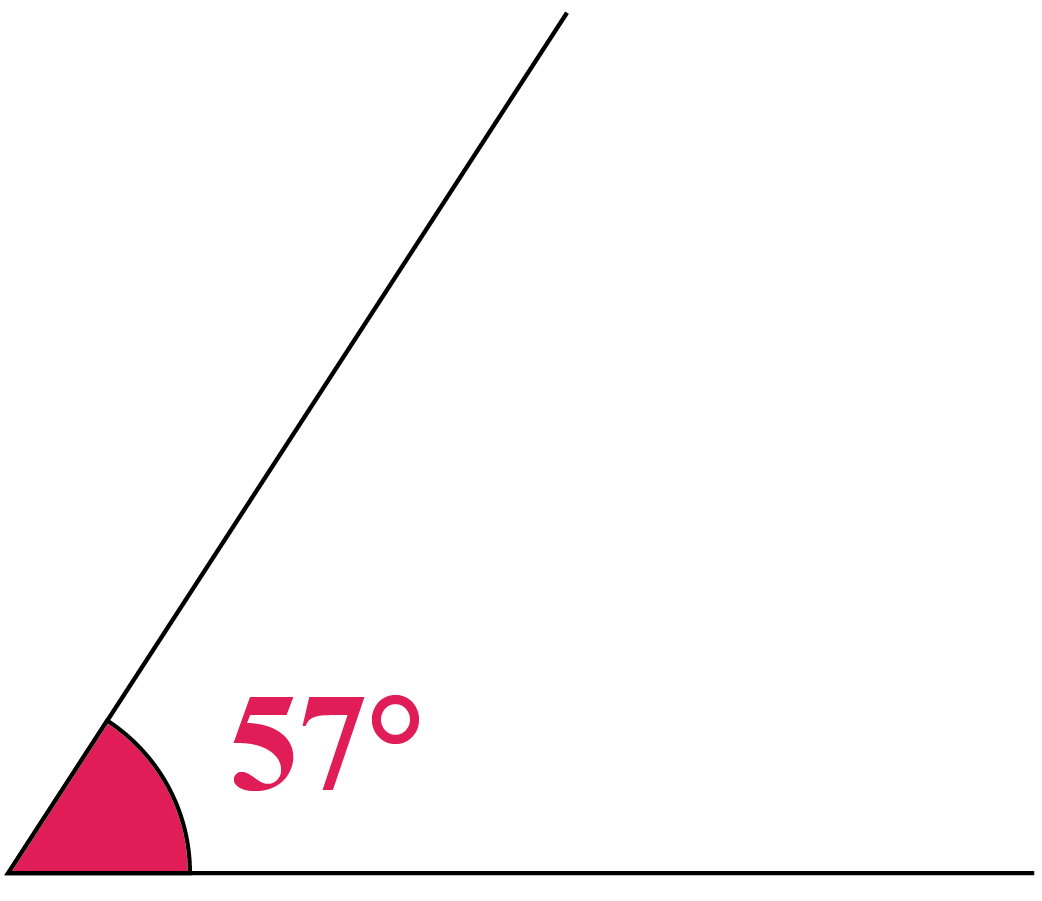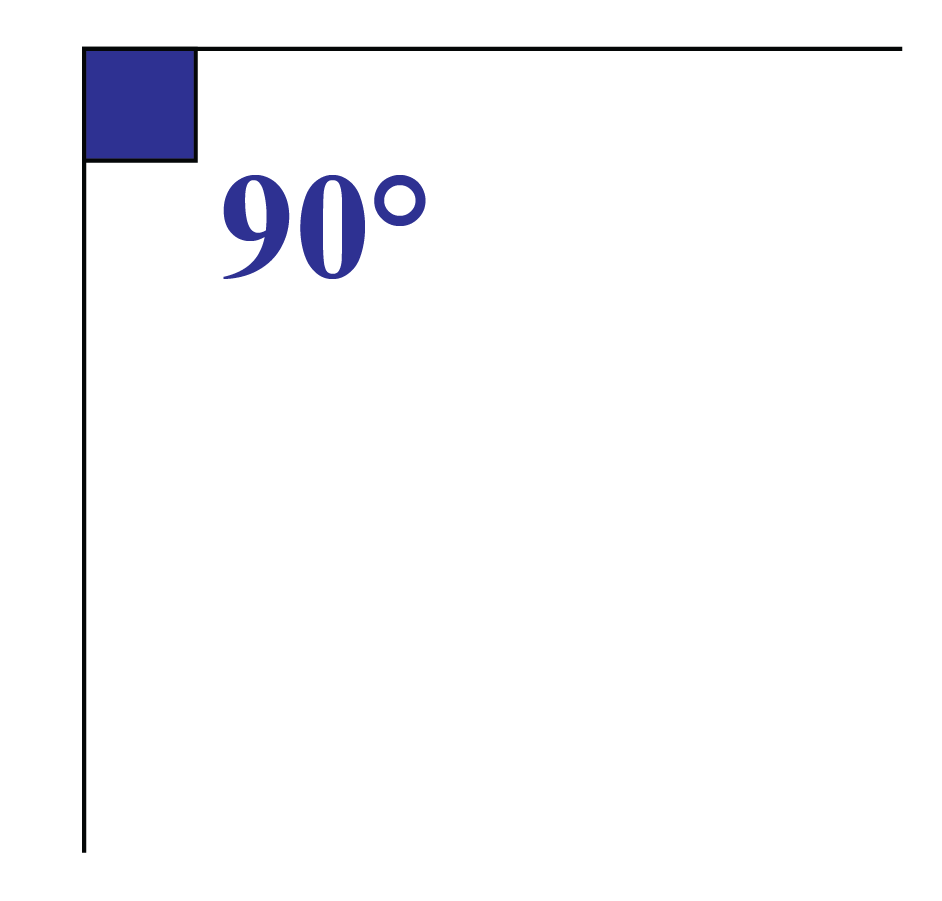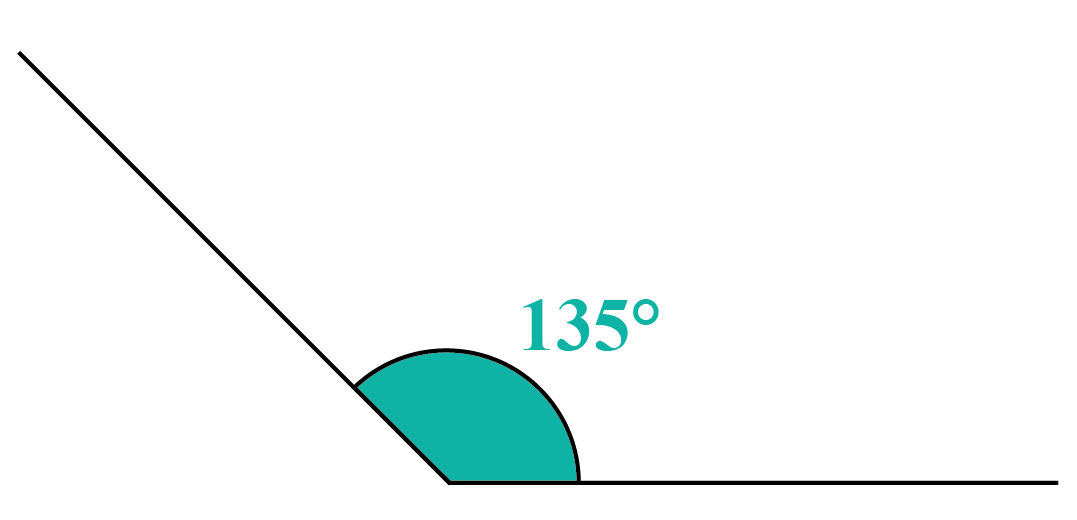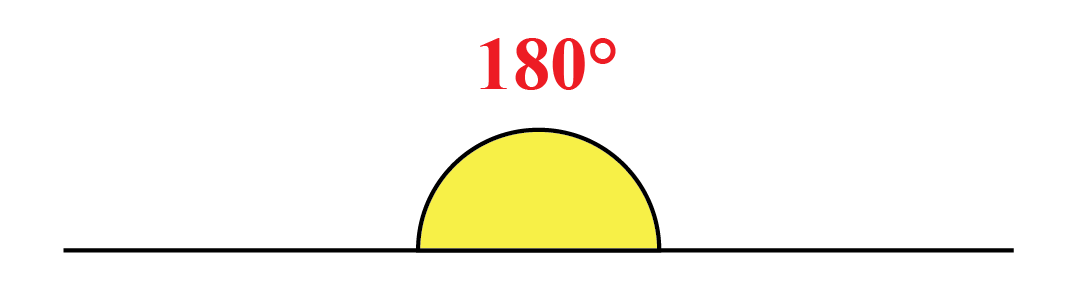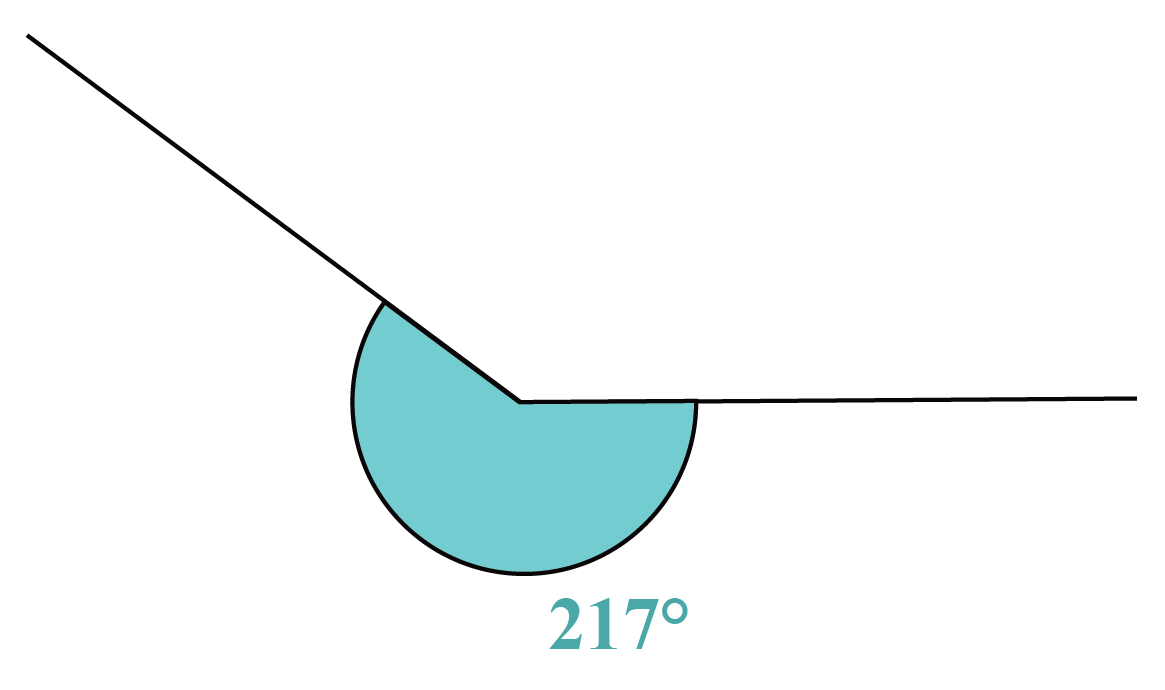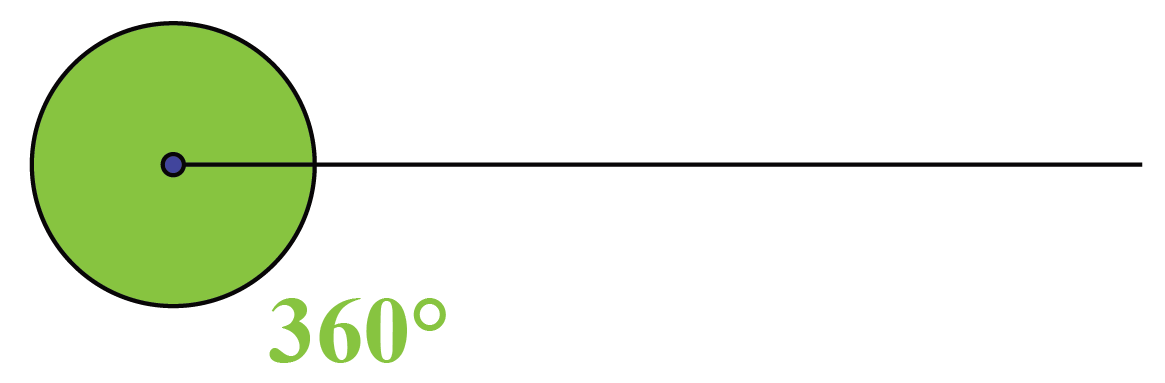There is one more place where the right angle is used and that is a right-angle triangle.

If among three angles of a triangle, one angle is 90°, then that triangle is called the right angle triangle.

Since the three interior angles of a right angle triangle add up to 180 degrees, and if one angle is always 90 degrees, then the other two angles must always add up to 90 degrees, and they are called complementary angles.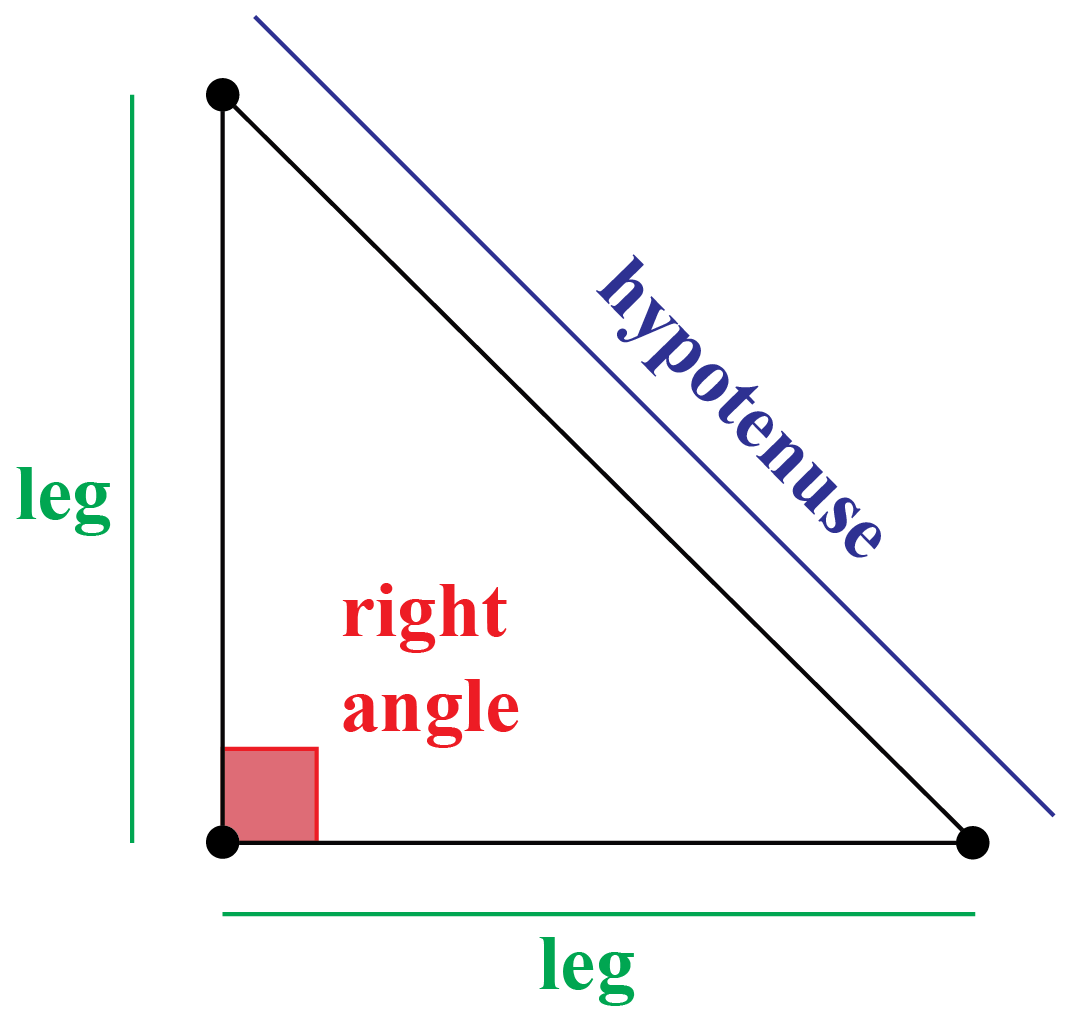Right angle triangle

More Important Topics
Numbers
Algebra
Geometry
Measurement
Money
Data
Trigonometry
Calculus